# Calculating Alpha Eigenvalues and Eigenfunctions with a Markov Transition Rate Matrix Monte Carlo Method

Nuclear Science and Engineering / Volume 192 / Number 2 / November 2018 / Pages 115-152

Technical Paper / dx.doi.org/10.1080/00295639.2018.1497397

For a nuclear system in which the entire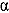-eigenvalue spectrum is known, eigenfunction expansion yields the time-dependent flux response to any arbitrary source. Applications in which this response is of interest include pulsed-neutron experiments, accelerator-driven subcritical systems, and fast burst reactors, where a steady-state assumption used in neutron transport is invalid for characterizing the time-dependent flux. To obtain the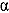-eigenvalue spectrum, the transition rate matrix method (TRMM) tallies transition rates describing neutron behavior in a discretized position-direction-energy phase space using Monte Carlo. Interpretation of the resulting Markov process transition rate matrix as the operator in the adjoint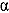-eigenvalue problem provides an avenue for determining a large finite set of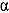eigenvalues and eigenfunctions of a nuclear system. Results from the TRMM are verified using analytic solutions, time-dependent Monte Carlo simulations, and modal expansion from diffusion theory. For simplified infinite-medium and one-dimensional geometries, the TRMM accurately calculates eigenvalues, eigenfunctions, and eigenfunction expansion solutions. Applications and comparisons to measurements are made for the small fast burst reactor CALIBAN and the Fort St. Vrain high-temperature gas-cooled reactor. For large three-dimensional geometries, discretization of the large position-energy-direction phase space limits the accuracy of eigenfunction expansion solutions using the TRMM, but it can still generate a fair estimate of the fundamental eigenvalue and eigenfunction. These results show that the TRMM generates an accurate estimate of a large number of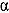eigenvalues. This is not possible with existing Monte Carlo–based methods.